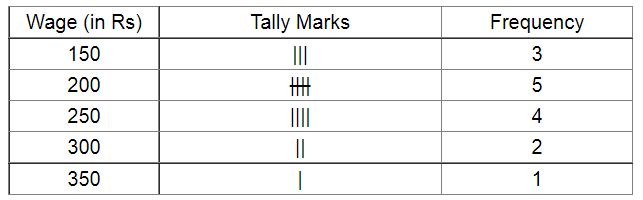# Following figures relate to the weekly wages (in Rs) of 15 workers in a factory:

Question:

Following figures relate to the weekly wages (in Rs) of 15 workers in a factory:

300, 250, 200, 250, 200, 150, 350, 200, 250, 200, 150, 300, 150, 200, 250

Prepare a frequency table.

(i) What is the range in wages (in Rs)?

(ii) How many workers are getting Rs 350?

(iii) How many workers are getting the minimum wages?

Solution:

The frequency table for the number of accidents per day for a period of 30 days is given below:(i) The range of wages (in Rs) is $350-150$ i.e. $200 .$

(ii) From the frequency table, we can see that the number of workers earning Rs 350 is 1.

(iii) Here, the minimum wage is 150. Hence, the number of workers earning the minimum wage is 3.